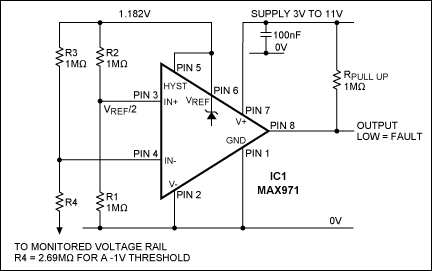# Precision Circuit Monitors Negative-Supply Thresholds

### Abstract

Using an IC (MAX971) that includes an open-drain comparator and a precision 1% voltage reference, this circuit monitors the magnitude of a negative supply voltage and provides a digital warning of fault conditions. To monitor multiple negative voltages, use the MAX974, which includes four comparators.

A similar version of this article appeared in the February 8, 2008 issue of Portable Design magazine.

A simple but accurate circuit (Figure 1) monitors the magnitude of a negative supply voltage. This capability is useful in multi-rail systems, particularly if the negative rail serves as the precision bias voltage for a GaAs device. The IC (MAX971) includes an open-drain comparator and a precision 1% voltage reference. Its trigger threshold is set by the value of external resistor R4. For convenience, all other resistors have the value 1MΩ.Resistors R1 and R2 divide the reference voltage, providing a trip point of Vref/2 for the comparator. R3 and R4 sample the negative voltage, and IC1 compares that sample to the trip-point voltage. R4 = 1MΩ + 2(1MΩ × VNEG/1.182V), where VNEG is the magnitude of the voltage being monitored (ignoring the minus sign). The circuit output goes low in response to a fault condition—i.e., when the magnitude of the monitored voltage drops below the set threshold. To ensure an overall accuracy better than 2%, all resistors should have 1% tolerance.

For monitoring more than one negative voltage, choose the MAX974, which includes four open-drain comparators with a precision 1% reference. Feed the output of R1 and R2 to all the comparators, add an R3/R4 pair for each monitored voltage, and calculate each R4 to yield a trip point for the corresponding voltage. All the open-drain outputs can be connected together.